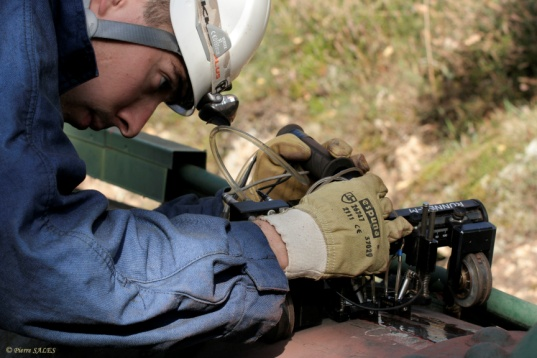EDF operates more than 450 hydroelectric plants that are supplied by 250 km of pressure pipes, aged of more than 60 years in average. Status diagnostics are regularly performed to control their mechanical strength. These diagnostics are based on a gathering of design and production data and on internal and external controls of the pressure pipe, e.g. thickness measurements. If the production data are insufficient, complementary investigations are performed on the material. Then, we calculate the margin factor of each section, equal to the ratio between the permissible stress and the maximal stress in use, depending on the residual thickness, the material characteristics and the maximal pressure in use.The margin factor value allows to define the fitness for service of each section. To consider the dispersions and uncertainties that affect the material characteristics and the residual thickness, the current practice consists in using the mean value minus two standard deviations for both magnitudes.

## Objectives and methods

The purpose of this analysis was to evaluate the level of reliability according to the margin factor value and to seek the multiplicative coefficients of the γ standard deviation that would allow to ensure a target probability of failure inferior to an annual target probability when the margin factor tends to 1.

Reliability computation

The FORM reliability method paired with the importance sampling simulation has been used to achieve the optimization of the safety coefficients.

Parametric analysis

The analysis consisted in varying the several input parameters (yield point, thickness, variation coefficient…) to calculate the reliability on a large sample of pipes.

Optimization

The optimization of the coefficients or the margin factor is realized by optimization under reliability constraints.

Graphic interface

In order to ease the use of the calculation technics, a version of Persalys has been adapted specifically for the study of pressure pipes.